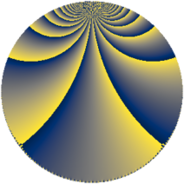# Properties

 Label 1216.4.zLevel $1216$ Weight $4$ Character orbit 1216.z Rep. character $\chi_{1216}(49,\cdot)$ Character field $\Q(\zeta_{12})$ Dimension $472$ Sturm bound $640$

# Related objects

## Defining parameters

 Level: $$N$$ $$=$$ $$1216 = 2^{6} \cdot 19$$ Weight: $$k$$ $$=$$ $$4$$ Character orbit: $$[\chi]$$ $$=$$ 1216.z (of order $$12$$ and degree $$4$$) Character conductor: $$\operatorname{cond}(\chi)$$ $$=$$ $$304$$ Character field: $$\Q(\zeta_{12})$$ Sturm bound: $$640$$

## Dimensions

The following table gives the dimensions of various subspaces of $$M_{4}(1216, [\chi])$$.

Total New Old
Modular forms 1952 488 1464
Cusp forms 1888 472 1416
Eisenstein series 64 16 48

## Trace form

 $$472q + 2q^{3} - 2q^{5} + O(q^{10})$$ $$472q + 2q^{3} - 2q^{5} + 8q^{11} - 2q^{13} - 236q^{15} - 4q^{17} - 20q^{19} + 52q^{21} + 116q^{27} - 2q^{29} - 1472q^{31} - 4q^{33} + 208q^{35} - 8q^{37} + 2q^{43} - 400q^{45} + 4q^{47} - 20792q^{49} + 638q^{51} - 2q^{53} + 2q^{59} - 1826q^{61} - 1368q^{63} - 16q^{65} + 1850q^{67} + 1996q^{69} + 6224q^{75} + 2736q^{77} - 8820q^{79} + 16520q^{81} - 2672q^{83} + 738q^{85} - 684q^{91} - 56q^{93} + 3872q^{95} - 4q^{97} + 1092q^{99} + O(q^{100})$$

## Decomposition of $$S_{4}^{\mathrm{new}}(1216, [\chi])$$ into newform subspaces

The newforms in this space have not yet been added to the LMFDB.

## Decomposition of $$S_{4}^{\mathrm{old}}(1216, [\chi])$$ into lower level spaces

$$S_{4}^{\mathrm{old}}(1216, [\chi]) \cong$$ $$S_{4}^{\mathrm{new}}(304, [\chi])$$$$^{\oplus 3}$$